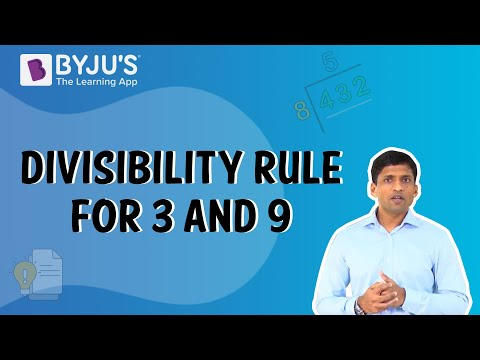# Playing With Numbers Class 8 Notes- Chapter 16

According to the CBSE Syllabus 2023-24, this chapter has been removed from NCERT Class 8 Maths textbook

## What is the General Form of Numbers?

If a two-digit number pq needs to represented in general form, then

pq=10p+q

To know more about Representing Numbers in General Form, visit here.

## Playing with 2 – Digit and 3 – Digit Numbers

### Numbers in General form

A two-digit number(ab) in its general form, is written as:ab=(10xa)+(1xb)

Similarly, a three-digit number (abc) is written as:

abc=(100xa)+(10xb)+c

### Reversing the 2 digit numbers and adding them

When a two-digit number is reversed and added to the number, the resulting number is perfectly divisible by 11 and the quotient is equal to the sum of the digits

For eg: The reverse of 29 is 92.

The sum of 29 and 92 = 29 +92 = 121.

On dividing the sum by 11, we get 121/11 = 11 = 9+2.

So, the sum is divisible by 11 and the quotient is equal to the sum of the digits of the number.

### Reversing the 2 digit numbers and Subtracting them

When a two-digit number is reversed and the larger number is subtracted from the smaller number, the resulting number is perfectly divisible by 9 and the quotient is equal to the difference of the digits of the number.

For example, the reverse of the number 39 is 93.

Now, 93 > 39.

So, 93 – 39 = 54

On dividing the difference of the two numbers by 9, we get, 54/9 = 6 = 9−3

So, the difference is divisible by 9 and the quotient is equal to the difference of the digits.

### Reversing the 3 digit numbers and Subtracting them

When a three-digit number is reversed and the smaller number is subtracted from the larger number, the resulting number is perfectly divisible by 99 and the quotient is equal to the difference between the first and third digit of the selected number.

For example, the reverse of 123 is 321.

Now, 321 > 123.

321 – 123 = 198

Now,198/99 = 2 = 3 – 1

So, the difference between 123 and 321 is divisible by 99 and the quotient is equal to the difference between 3 and 1.

### Taking all the combinations of 3 digit numbers and adding them

If all the combinations of a three-digit number are taken and added together, then the resulting number is perfectly divisible by 111.

Let us take 123.

The various numbers that can be formed using the digits of 123 are 123, 132, 213, 231, 312 and 321.

The sum of these numbers is equal to (123 + 132 + 213 + 231 + 312 + 321 = 1332)

Now,1332/111 = 12

So, the sum of all the combinations is divisible by 111.

## Puzzles with Digits

### Letters for digits

Here we have puzzles in which letters take the place of digits in an arithmetic ‘sum’, and the problem is to find out which letter represents which digit.

For example, if :

4 Q 1

3 8 Q

——— (+)

8 0 3

———

Then the value of Q will be 2 as 2 is the only digit which results in 3 when 1 is added to it. Also, 2+8 =10, 1 as carry and then 1+4+3 =8. So, the value of Q is 2.

To know more about Puzzles Involving Numbers, visit here.

## Why Divisibility Rules?

### Divisibility by 10

If the units digit of a number is 0 then the number is divisible by 10.

For example, 1390 is divisible by 10. 113 is not divisible by 10 because it has 3 in its units place.

### Divisibility by 5

If the units digit of a number is either 5 or 0 then it isdivisible by 5.

For example, 135 is divisible by 5 but 133 is not divisible by 5.

### Divisibility by 2

If the units digit of a number is 0,2,4,6 or 8, then it is divisible by 2.

For example 22, 24, 68 are divisible by 2 but 23, 33, 45 are not.

### Divisibility by 3

A number is divisible by 3 if thesum of its digits is divisible by 3.

For eg:The sum of digits of 144 = 1 + 4 + 4 = 9, which is divisible by 3. So, 144 is divisible by 3.

The sum of digits of 266 = 2 + 6 + 6 = 14, which is not divisible by 3. So, 266 is not divisible by 3.

### Divisibility by 9

A number is divisible by 9 if the sum of its digits is divisible by 9.

For example, the sum of digits of 277 = 2 + 7 + 7 = 16, which is not divisible by 9. So, 277 is not divisible by 9.

The sum of digits of 783 = 7 + 8 + 3 = 18, which is divisible by 9. So, 783 is divisible by 9.

#### For more information on Divisibility Rule For 3 And 9, watch the below video.To know more about Divisibility Rules, visit here.

## Frequently Asked Questions on CBSE Class 8 Maths Notes Chapter 16 Playing with Numbers

Q1

### What are Prime numbers?

Prime numbers are numbers that have only 2 factors: 1 and the number itself.

Q2

### What is Divisibility test?

A divisibility test is an easy way of finding out whether a given integer is divisible by a fixed divisor without executing the division, usually by examining its digits

Q3

### What are Common factors?

A common factor is a number that can be divided into two different numbers, without leaving a remainder.